The GLIMMIX Procedure
 CONTRAST Statement
CONTRAST ’label’ contrast-specification
<, contrast-specification> <, ...>
< / options>
;

The CONTRAST statement provides a mechanism for obtaining custom hypothesis tests. It is patterned after the CONTRAST statement in PROC MIXED and enables you to select an appropriate inference space (McLean, Sanders, and Stroup 1991). The GLIMMIX procedure gives you greater flexibility in entering contrast coefficients for random effects, however, because it permits the usual value-oriented positional syntax for entering contrast coefficients, as well as a level-oriented syntax that simplifies entering coefficients for interaction terms and is designed to work with constructed effects that are defined through the experimental EFFECT statement. The differences between the traditional and new-style coefficient syntax are explained in detail in the section Positional and Nonpositional Syntax for Contrast Coefficients.

You can test the hypothesis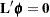, where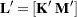and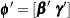, in several inference spaces. The inference space corresponds to the choice of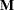. When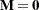, your inferences apply to the entire population from which the random effects are sampled; this is known as the broad inference space. When all elements ofare nonzero, your inferences apply only to the observed levels of the random effects. This is known as the narrow inference space, and you can also choose it by specifying all of the random effects as fixed. The GLM procedure uses the narrow inference space. Finally, by zeroing portions ofcorresponding to selected main effects and interactions, you can choose intermediate inference spaces. The broad inference space is usually the most appropriate; it is used when you do not specify random effects in the CONTRAST statement.

In the CONTRAST statement,

label

identifies the contrast in the table. A label is required for every contrast specified. Labels can be up to 200 characters and must be enclosed in quotes.

contrast-specification

identifies the fixed effects and random effects and their coefficients from which thematrix is formed. The syntax representation of a contrast-specification is
< fixed-effect values ...> < | random-effect values ...>

fixed-effect

identifies an effect that appears in the MODEL statement. The keyword INTERCEPT can be used as an effect when an intercept is fitted in the model. You do not need to include all effects that are in the MODEL statement.

random-effect

identifies an effect that appears in the RANDOM statement. The first random effect must follow a vertical bar (|); however, random effects do not have to be specified.

values

are constants that are elements of thematrix associated with the fixed and random effects. There are two basic methods of specifying the entries of thematrix. The traditional representation—also known as the positional syntax—relies on entering coefficients in the position they assume in thematrix. For example, in the following statements the elements ofassociated with the b main effect receive ain the first position and a
in the second position:

```class a b;
model y = a b a*b;
contrast 'B at A2' b 1 -1  a*b 0  0  1 -1;
```

The elements associated with the interaction receive ain the third position and ain the fourth position. In order to specify coefficients correctly for the interaction term, you need to know how the levels of a and b vary in the interaction, which is governed by the order of the variables in the CLASS statement. The nonpositional syntax is designed to make it easier to enter coefficients for interactions and is necessary to enter coefficients for effects constructed with the experimental EFFECT statement. In square brackets you enter the coefficient followed by the associated levels of the CLASS variables. If B has two and A has three levels, the previous CONTRAST statement, by using nonpositional syntax for the interaction term, becomes

```contrast 'B at A2' b 1 -1 a*b [1, 2 1] [-1, 2 2];
```

It assigns valueto the interaction where A is at level 2 and B is at level 1, and it assignsto the interaction where both classification variables are at level 2. The comma separating the entry for thematrix from the level indicators is optional. Further details about the nonpositional contrast syntax and its use with constructed effects can be found in the section Positional and Nonpositional Syntax for Contrast Coefficients. Nonpositional syntax is available only for fixed-effects coefficients.

The rows of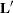are specified in order and are separated by commas. The rows of the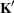component ofare specified on the left side of the vertical bars (|). These rows test the fixed effects and are, therefore, checked for estimability. The rows of the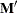component ofare specified on the right side of the vertical bars. They test the random effects, and no estimability checking is necessary.

If PROC GLIMMIX finds the fixed-effects portion of the specified contrast to be nonestimable (see the SINGULAR= option), then it displays missing values for the test statistics.

If the elements ofare not specified for an effect that contains a specified effect, then the elements of the unspecified effect are automatically "filled in" over the levels of the higher-order effect. This feature is designed to preserve estimability for cases where there are complex higher-order effects. The coefficients for the higher-order effect are determined by equitably distributing the coefficients of the lower-level effect as in the construction of least squares means. In addition, if the intercept is specified, it is distributed over all classification effects that are not contained by any other specified effect. If an effect is not specified and does not contain any specified effects, then all of its coefficients inare set to 0. You can override this behavior by specifying coefficients for the higher-order effect.

If too many values are specified for an effect, the extra ones are ignored; if too few are specified, the remaining ones are set to 0. If no random effects are specified, the vertical bar can be omitted; otherwise, it must be present. If a SUBJECT effect is used in the RANDOM statement, then the coefficients specified for the effects in the RANDOM statement are equitably distributed across the levels of the SUBJECT effect. You can use the E option to see exactly whatmatrix is used.

PROC GLIMMIX handles missing level combinations of classification variables similarly to PROC GLM and PROC MIXED. These procedures delete fixed-effects parameters corresponding to missing levels in order to preserve estimability. However, PROC MIXED and PROC GLIMMIX do not delete missing level combinations for random-effects parameters, because linear combinations of the random-effects parameters are always estimable. These conventions can affect the way you specify your CONTRAST coefficients.

The CONTRAST statement computes the statistic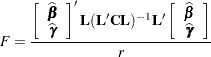where, and approximates its distribution with an F distribution unless DDFM=NONE. If you select DDFM=NONE as the degrees-of-freedom method in the MODEL statement, and if you do not assign degrees of freedom to the contrast with the DF= option, then PROC GLIMMIX computes the test statistic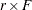and approximates its distribution with a chi-square distribution. In the expression for,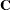is an estimate of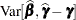; see the section Estimated Precision of Estimates and the section Aspects Common to Adaptive Quadrature and Laplace Approximation for details about the computation ofin a generalized linear mixed model.

The numerator degrees of freedom in the F approximation and the degrees of freedom in the chi-square approximation are equal to. The denominator degrees of freedom are taken from the "Tests of Fixed Effects" table and correspond to the final effect you list in the CONTRAST statement. You can change the denominator degrees of freedom by using the DF= option.

You can specify the following options in the CONTRAST statement after a slash (/).

BYCATEGORY
BYCAT

requests that in models for nominal data (generalized logit models) the contrasts not be combined across response categories but reported separately for each category. For example, assume that the response variable Style is multinomial with three (unordered) categories. The following GLIMMIX statements fit a generalized logit model relating the preferred style of instruction to school and educational program effects:

```proc glimmix data=school;
class School Program;
model Style(order=data) = School Program / s ddfm=none
freq Count;
contrast 'School 1 vs. 2' school 1 -1;
contrast 'School 1 vs. 2' school 1 -1 / bycat;
run;
```

The first contrast compares school effects in all categories. This is a two-degrees-of-freedom contrast because there are two nonredundant categories. The second CONTRAST statement produces two single-degree-of-freedom contrasts, one for each nonreference Style category.

The BYCATEGORY option has no effect unless your model is a generalized (mixed) logit model.

CHISQ

requests that chi-square tests be performed for all contrasts in addition to any F tests. A chi-square statistic equals its corresponding F statistic times the numerator degrees of freedom, and these same degrees of freedom are used to compute the p-value for the chi-square test. This p-value will always be less than that for thetest, because it effectively corresponds to antest with infinite denominator degrees of freedom.

DF=number

specifies the denominator degrees of freedom for the F test. For the degrees of freedom methods DDFM=BETWITHIN, DDFM=CONTAIN, and DDFM=RESIDUAL, the default is the denominator degrees of freedom taken from the "Tests of Fixed Effects" table and corresponds to the final effect you list in the CONTRAST statement. For DDFM=NONE, infinite denominator degrees of freedom are assumed by default, and for DDFM=SATTERTHWAITE and DDFM=KENWARDROGER, the denominator degrees of freedom are computed separately for each contrast.

E

requests that thematrix coefficients for the contrast be displayed.

GROUP coeffs

sets up random-effect contrasts between different groups when a GROUP= variable appears in the RANDOM statement. By default, CONTRAST statement coefficients on random effects are distributed equally across groups. If you enter a multiple row contrast, you can also enter multiple rows for the GROUP coefficients. If the number of GROUP coefficients is less than the number of contrasts in the CONTRAST statement, the GLIMMIX procedure cycles through the GROUP coefficients. For example, the following two statements are equivalent:

```contrast 'Trt 1 vs 2 @ x=0.4' trt 1 -1  0 | x 0.4,
trt 1  0 -1 | x 0.4,
trt 1 -1  0 | x 0.5,
trt 1  0 -1 | x 0.5 /
group 1 -1, 1 0 -1, 1 -1, 1 0 -1;

contrast 'Trt 1 vs 2 @ x=0.4' trt 1 -1  0 | x 0.4,
trt 1  0 -1 | x 0.4,
trt 1 -1  0 | x 0.5,
trt 1  0 -1 | x 0.5 /
group 1 -1, 1 0 -1;
```
SINGULAR=number

tunes the estimability checking. Ifis a vector, define ABS() to be the largest absolute value of the elements of. If ABS(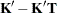) is greater than*number for any row ofin the contrast, then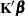is declared nonestimable. Here,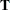is the Hermite form matrix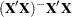, andis ABS(), except when it equals 0, and thenis 1. The value for number must be between 0 and 1; the default is 1E4.

SUBJECT coeffs

sets up random-effect contrasts between different subjects when a SUBJECT= variable appears in the RANDOM statement. By default, CONTRAST statement coefficients on random effects are distributed equally across subjects. Listing subject coefficients for multiple row CONTRAST statements follows the same rules as for GROUP coefficients.Previous Page | Next Page | Top of Page